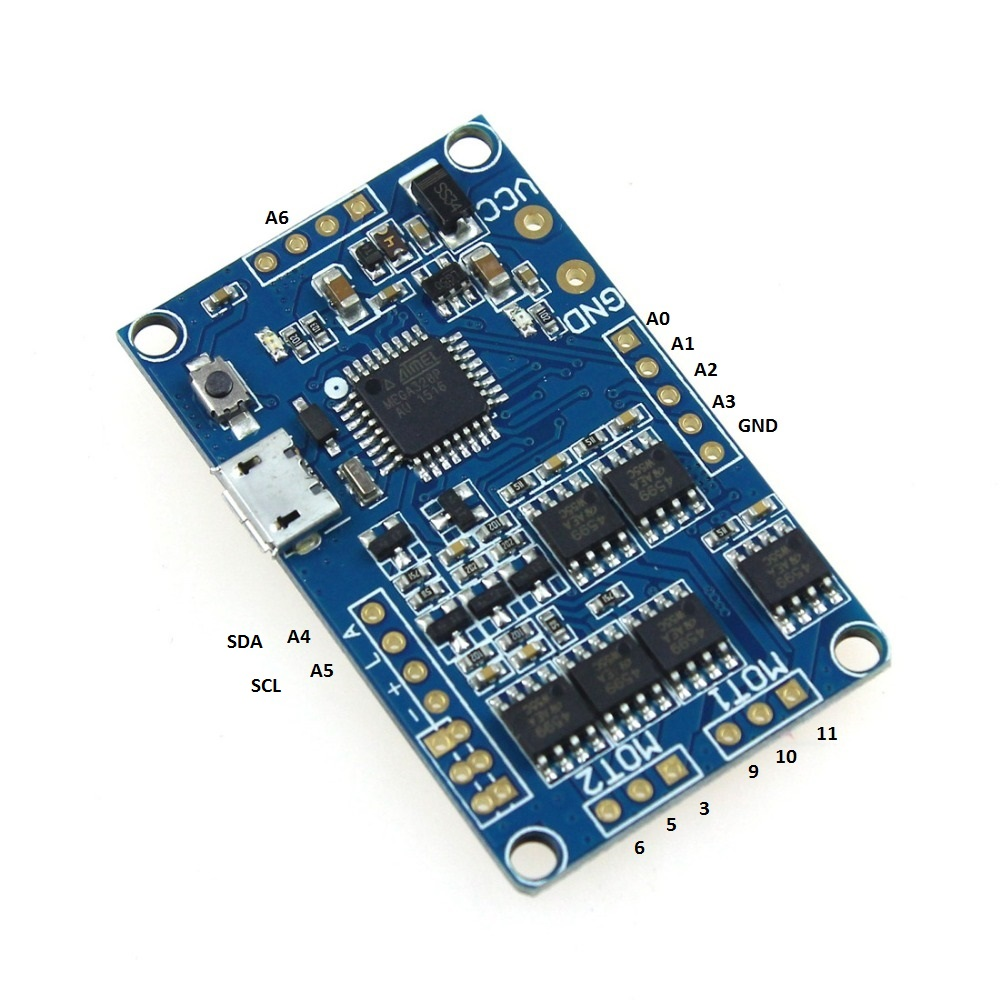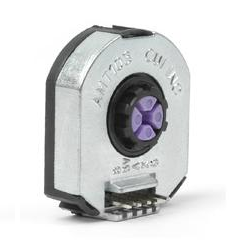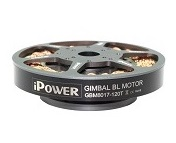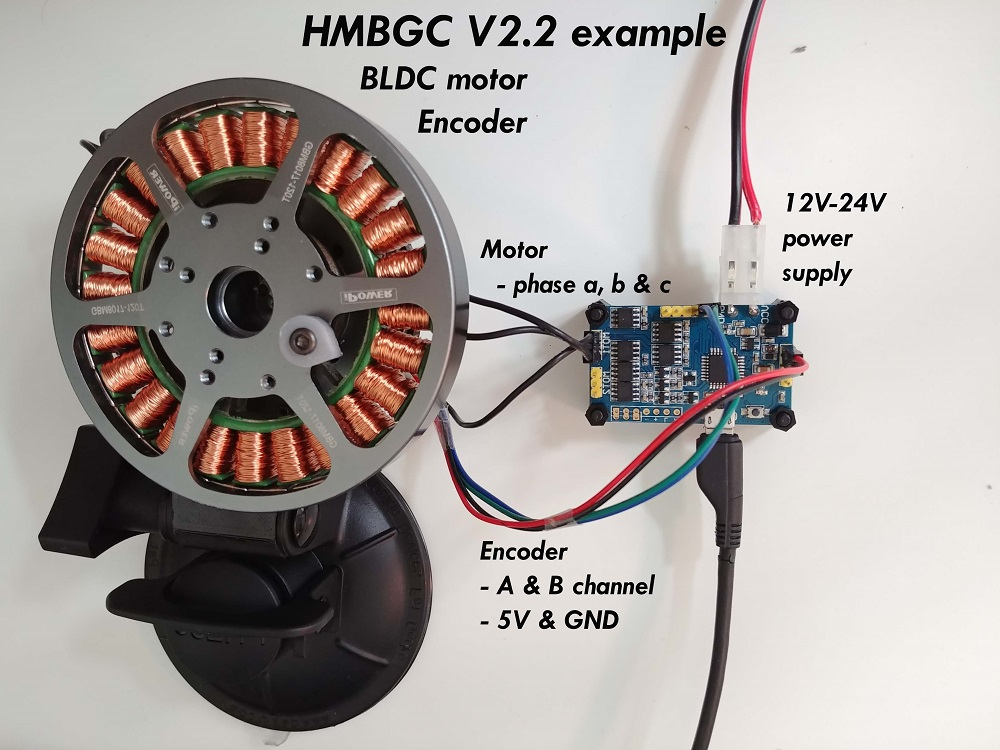Velocity control exampleusing HMBGC V2.2 board

This is a very simple and cool example of using the FOC algorithm using the gimbal controller board. They are not meant to be used with the closed loop position control but SimpleFOClibrary makes it not just possible but also pretty simple.

Here is the hardware we used for this project:

HMBGC V2.2AMT 103 encoderIPower GBM8017-120TConnecting everything together

For a bit more in depth explanation of HMBGC V2.2 connections please check the connection examples.Encoder

Pinout restriction

HMBGC doesn't have access to the Arduino's external interrupt pins 2 and 3, moreover the only pins we have access to are analog pins A0-A7. Therefore we need to read the encoder channels using the software interrupt library, please check the encoder code implementation for more information.
• Encoder channels A and B are connected to the pins A0 and A1.

Motor

• Motor phases a,b and c are connected directly to the driver outputs
• Motor terminal M1 uses Arduino pins 9,10,11 and M2 uses 3,5,6

Arduino code

Let’s go through the full code for this example and write it together. First thing you need to do is include the SimpleFOC library:

#include <SimpleFOC.h>

Make sure you have the library installed. If you still don’t have it please check the get started page.

Also in the case of the gimbal controllers like the HMBGC we do not have access to the hardware interrupt pins so you will need to have a software interrupt library. I would suggest using PciManager library. If you have not installed it yet, you can do it using the Arduino library manager directly. Please check the Encoder class docs for more info. So once you have it please include it to the sketch:

// software interrupt library
#include <PciManager.h>
#include <PciListenerImp.h>

Encoder code

First, we define the Encoder class with the A and B channel pins and number of impulses per revolution.

// define Encoder
Encoder encoder = Encoder(A0, A1, 2048);

Then we define the buffering callback functions.

// channel A and B callbacks
void doA(){encoder.handleA();}
void doB(){encoder.handleB();}

Next we define the PciManager pin change listeners:

// pin change listeners
PciListenerImp listenerA(encoder.pinA, doA);
PciListenerImp listenerB(encoder.pinB, doB);

In the setup() function first we initialize the encoder:

// initialize encoder hardware
encoder.init();

And then instead of calling encoder.enableInterrupt() function we use the PciManager library interface to attach the interrupts.

// interrupt initialization
PciManager.registerListener(&listenerA);
PciManager.registerListener(&listenerB);

And that is it, let’s setup the motor.

For more configuration parameters of the encoders please check the Encoder class docs.

Motor code

First we need to define the BLDCMotor class with the number od pole pairs (14)

// define BLDC motor
BLDCMotor motor = BLDCMotor(14);
If you are not sure what your pole pairs number is please check the find_pole_pairs.ino example.

Next we need to define the BLDCDriver3PWM class with the PWM pin numbers of the motor

// define BLDC driver
BLDCDriver3PWM driver = BLDCDriver3PWM(9, 10, 11);

Then in the setup() we configure first the voltage of the power supply if it is not 12 Volts and init the driver.

// power supply voltage
// default 12V
driver.voltage_power_supply = 12;
driver.init();

Then we tell the motor which control loop to run by specifying the motor.controller variable.

// set control loop type to be used
// MotionControlType::torque
// MotionControlType::velocity
// MotionControlType::angle
motor.controller = MotionControlType::velocity;

Now we configure the PI controller parameters

// velocity PI controller parameters
// default P=0.5 I = 10
motor.PID_velocity.P = 0.2;
motor.PID_velocity.I = 20;
// jerk control using voltage voltage ramp
// default value is 300 volts per sec  ~ 0.3V per millisecond
motor.PID_velocity.output_ramp = 1000;

//default voltage_power_supply
motor.voltage_limit = 6;

Additionally we can configure the Low pass filter time constant Tf

// velocity low pass filtering
// default 5ms - try different values to see what is the best.
// the lower the less filtered
motor.LPF_velocity.Tf = 0.01;

And finally we connect the encoder and the driver to the motor, do the hardware init and init of the Field Oriented Control.

// link the motor to the sensor

// initialize motor
motor.init();
// align encoder and start FOC
motor.initFOC();

The last peace of code important for the motor is of course the FOC routine in the loop function.

void loop() {
// iterative FOC function
motor.loopFOC();

// iterative function setting and calculating the velocity loop
// this function can be run at much lower frequency than loopFOC function
motor.move(target_velocity);
}

That is it, let’s see the full code now!

For more configuration parameters and control loops please check the BLDCMotor class doc.

Full Arduino code

To the full code I have added a small serial commander interface, to be able to change velocity target value in real time.

#include <SimpleFOC.h>
// software interrupt library
#include <PciManager.h>
#include <PciListenerImp.h>

// define BLDC motor
BLDCMotor motor = BLDCMotor( 14 );
// define driver
BLDCDriver3PWM driver = BLDCDriver3PWM(9, 10, 11);
//  define Encoder
Encoder encoder = Encoder(A0, A1, 500);
// interrupt routine initialization
void doA(){encoder.handleA();}
void doB(){encoder.handleB();}

// encoder interrupt init
PciListenerImp listenerA(encoder.pinA, doA);
PciListenerImp listenerB(encoder.pinB, doB);

// target variable
float target_velocity=0;
// commander interface
Commander command = Commander(Serial);
void onTarget(char* cmd){ command.scalar(&target_velocity, cmd); }

void setup() {
// initialize encoder hardware
encoder.init();
// interrupt initialization
PciManager.registerListener(&listenerA);
PciManager.registerListener(&listenerB);
// link the motor to the sensor

// power supply voltage
// default 12V
driver.voltage_power_supply = 12;
driver.init();
// link the motor to the driver

// set FOC loop to be used
// MotionControlType::torque
// MotionControlType::velocity
// MotionControlType::angle
motor.controller = MotionControlType::velocity;

// controller configuration based on the control type
// velocity PI controller parameters
// default P=0.5 I = 10
motor.PID_velocity.P = 0.2;
motor.PID_velocity.I = 20;
// jerk control using voltage voltage ramp
// default value is 300 volts per sec  ~ 0.3V per millisecond
motor.PID_velocity.output_ramp = 1000;

// velocity low pass filtering
// default 5ms - try different values to see what is the best.
// the lower the less filtered
motor.LPF_velocity.Tf = 0.01;

//default voltage_power_supply
motor.voltage_limit = 6;

// initialize motor
motor.init();
// align encoder and start FOC
motor.initFOC();

// monitoring port
Serial.begin(115200);
Serial.println("Set the target velocity using serial terminal:");
_delay(1000);
}

void loop() {
// iterative FOC function
motor.loopFOC();

// 0.5 hertz sine wave
//target_velocity = sin( micros()*1e-6 *2*M_PI * 0.5 );
motor.move(target_velocity);

// iterative function setting the velocity target
command.run();
}